# Sampling with replacement until you’ve seen everything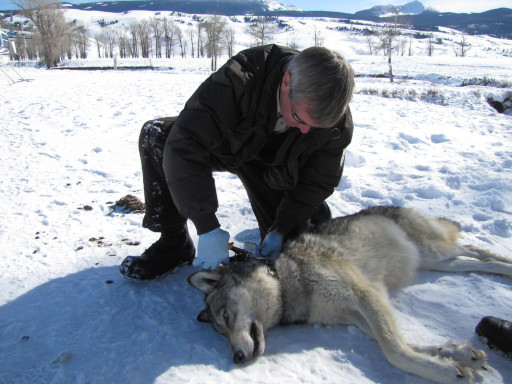Suppose you have a standard deck of 52 cards. You pull out a card, put it back in the deck, shuffle, and pull out another card. How long would you expect to do this until you’ve seen every card?

Here’s a variation on the same problem. Suppose you’re a park ranger keeping data on tagged wolves in a national park. Each day you catch a wolf at random, collect some data, and release it. If there are 100 wolves in the park, how long until you’ve seen every wolf at least once?

We’ll solve this problem via simulation, then exactly.

## Simulation

The Python code to solve the problem via simulation is straight forward. Here we’ll simulate our ranger catching and releasing one of 100 wolves. We’ll repeat our simulation five times to get an idea how much the results vary.

    import numpy as np
from numpy.random import default_rng

rng = default_rng()
num_runs = 5
N = 100 # number of unique items

for run in range(num_runs):
tally = np.zeros(N,dtype=int)
trials = 0
while min(tally) == 0:
tally[rng.integers(N)] += 1
trials += 1
print(trials)


When I ran this, I got 846, 842, 676, 398, and 420.

## Exact solution

Suppose we have a desk of N cards, and we sample the deck with replacement M times. We can select the first card N ways. Ditto for the second, third, and all the rest of the cards. so there are NM possible sequences of card draws.

How many of these sequences include each card at least once? To answer the question, we look at Richard Stanley’s twelvefold way. We’re looking for the number of ways to allocate M balls into N urns such that each urn contains at least one ball. The answer is

N! S(M, N)

where S(M, N) is the Stirling number of the second kind with parameters M and N .

So the probability of having seen each card at least once after selecting M cards randomly with replacement is

N! S(M, N) / NM.

## Computing Stirling numbers

We’ve reduced our problem to the problem of computing Stirling numbers (of the second kind), so how do we do that?

We can compute Stirling numbers in terms of binomial coefficients as follows.Now we have a practical problem if we want to use this formula for larger values of n and k. If we work with floating point numbers, we’re likely to run into overflow. This is the perennial with probability calculations. You may need to compute a moderate-sized number as the ratio of two enormous numbers. Even though the final result is between 0 and 1, the intermediate results may be too large to store in a floating point number. And even if we don’t overflow, we may lose precision because we’re working with an alternating sum.

The usual way to deal with overflow is to work on a log scale. But that won’t work for Stirling numbers because we’re adding terms together, not multiplying them.

One way to solve our problem—not the most efficient way but a way that will work—is to work with integers. This is easy in Python because integers by default can be arbitrarily large.

There are two ways to compute binomial coefficients in Python: scipy.special.binom and math.comb. The former uses floating point operations and the latter uses integers, so we need to use the latter.

We can compute the numerator of our probability with

    from math import comb
def k_factorial_stirling(n, k):
return sum((-1)**i * comb(k, i)*(k-i)**n for i in range(k+1))


Then our probability is

    k_factorial_stirling(M, N) / N**M

If we compute the probability that our ranger has seen all 100 wolves after catching and releasing 500 times, we get 0.5116. So the median number of catch and release cycles is somewhere around 500, which is consistent with our simulation above.

Note that the numerator and denominator in our calculation are both on the order of 101000 while the largest floating point number is on the order of 10308, so we would have overflowed if we’d used binom instead of comb.

## Related posts

 It’s unfortunate that these were called the “second kind” because they’re the kind that come up most often in application.

# How flat is a normal mixture on top?

Male and female heights both have a standard deviation of about 3 inches, with means of 70 inches and 64 inches. That’s a good first-pass model using round numbers.

If you ask what the height of an average adult is, not specifying male or female, you get a mixture of two normal distributions. If we assume an equal probability of selecting a male or female, then the probability density function for the mixture is the average of the density functions for men and women separately.

This mixture distribution is remarkably flat on top.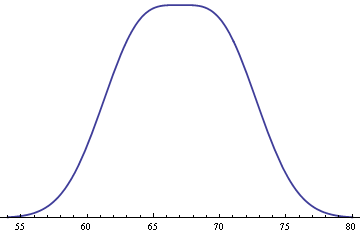This happens whenever you have an equal mixture of two normal distributions, both having the same standard deviation σ, and with means 2σ apart. If the means were any closer together, the distribution would be rounder on top. If the means were any further apart, there would be a dip in the middle.

This makes sense intuitively if you think about what happens if you make things more extreme. If the means were as close together as possible, i.e. if they were the same, we’d simply have a normal distribution with its familiar curve on top. If the means were much further apart, we’d have two bumps that hardly appear to overlap.

See more on the application to heights here.

## Mathematical details

How flat is the mixture density on top? We can quantify the flatness by looking at a power series expansion centered at the peak.

To simplify matters, we can assume that σ = 1 and that the means of the two distributions are -μ and μ, with the primary interest being the case μ = 1. The probability density function for the mixture isIf we expand this in a Taylor series around 0 we getThis is a little complicated, but it explains a lot. Notice that the coefficient of x² has a term (μ² – 1).

We said above that if the means were any closer together than two standard deviations, the distribution would be rounder on top. That’s because if μ < 1 then (μ² – 1) is negative and the curve is convex on top.

We also said that if the means were any further apart, there would be a dip in the middle. That’s because if μ > 1 then (μ² – 1) is positive and the curve is concave on top.

Now if μ = 1 then the x² term disappears. And because our function is even, its Taylor series only has terms with even powers. So if the x² term goes away the next term is not x3 but x4.

So one way to say how flat our curve is on top is that it is flat to within O(x4).

Let’s get more specific by evaluating the coefficients numerically. For small x, our mixture density function is

0.0965 – 0.0080 x4 + O(x6).

Now if x is small, x4 is extremely small, and the coefficient of 0.008 makes it even smaller.

# Order statistics for normal distributions

The last couple posts have touched on order statistics for normal random variables. I wrote the posts quickly and didn’t go into much detail, and more detail would be useful.

Given two integers nr ≥ 1, define E(r, n) to be the rth order statistic of n samples from standard normal random variables. That is, if we were to take n samples and then sort them in increasing order, the expected value of the rth sample is E(r, n).

We can compute E(r, n) exactly bywhere φ and Φ are the PDF and CDF of a standard normal random variable respectively. We can numerically evaluate E(r, n) by numerically evaluating its defining integral.

The previous posts have used

dn = E(n, n) – E(1, n) = 2 E(n, n).

The second equality above follows by symmetry.

We can compute dn in Mathematica as follows.

    Phi[x_] := (1 + Erf[x/Sqrt])/2
phi[x_] := Exp[-x^2/2]/Sqrt[2 Pi]
d[n_] := 2 n NIntegrate[
x Phi[x]^(n - 1) phi[x], {x, -Infinity, Infinity}]

And we can reproduce the table here by

    Table[1/d[n], {n, 2, 10}]

Finally, we can see how dn behaves for large n by calling

    ListPlot[Table[d[n], {n, 1, 1000}]]

to produce the following graph.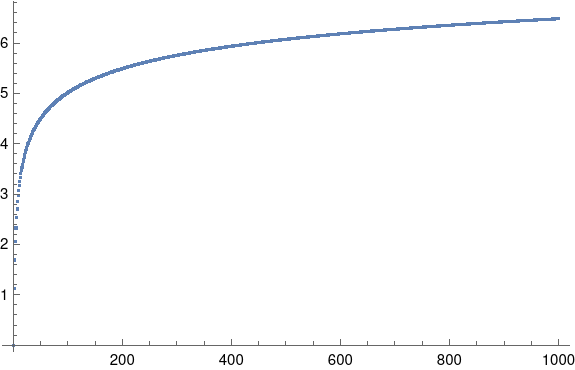See the next post for approximations to dn.

# Range trick for larger sample sizes

The previous post showed that the standard deviation of a sample of size n can be well estimated by multiplying the sample range by a constant dn that depends on n.

The method works well for relatively small n. This should sound strange: typically statistical methods work better for large samples, not small samples. And indeed this method would work better for larger samples. However, we’re not so much interested in the efficiency of the method per se, but its efficiency relative to the standard way of estimating standard deviation. For small samples, both methods are not very accurate, and the two methods appear to work equally well.

If we want to use the range method for larger n, there are a couple questions: how well does the method work, and how do we calculate dn.

## Simple extension

Ashley Kanter left a comment on the previous post saying

dn = 3 log n0.75 (where the log is base 10) seems to perform quite well even for larger n.

Ashley doesn’t say where this came from. Maybe it’s an empirical fit.

The constant dn is the expected value of the range of n samples from a standard normal random variable. You could find a (complicated) expression for this and then find a simpler expression using an asymptotic approximation. Maybe I’ll try that later, but for now I need to wrap up this post and move on to client work.

Note that

log10 n0.75 = 0.977 loge(n)

and so we could use

dn = log n

where log is natural log. This seems like something that might fall out of an asymptotic approximation. Maybe Ashley empirically discovered the first term of a series approximation.

Update: See this post for a more detailed exploration of how well log n, square root of n, and another method approximate dn.

Update 2: Ashley Kanter’s approximation was supposed to be 3 (log10 n) 0.75 rather than 3 log10 (n0.75) and is a very good approximation. This is also addressed in the link in the first update.

## Simulation

Here’s some Python code to try things out.

    import numpy as np
from scipy.stats import norm

np.random.seed(20220309)

n = 20
for _ in range(5):
x = norm.rvs(size=n)
w = x.max() - x.min()
print(x.std(ddof=1), w/np.log(n))


And here are the results.

    |   std | w/d_n |
|-------+-------|
| 0.930 | 1.340 |
| 0.919 | 1.104 |
| 0.999 | 1.270 |
| 0.735 | 0.963 |
| 0.956 | 1.175 |


It seems the range method has more variance, though notice in the fourth row that the standard estimate can occasionally wander pretty far from the theoretical value of 1 as well.

We get similar results when we increase n to 50.

    |   std | w/d_n |
|-------+-------|
| 0.926 | 1.077 |
| 0.889 | 1.001 |
| 0.982 | 1.276 |
| 1.038 | 1.340 |
| 1.025 | 1.209 |


## Not-so-simple extension

There are ways to improve the range method, if by “improve” you mean make it more accurate. One way is to divide the sample into random partitions, apply the method to each partition, and average the results. If you’re going to do this, partitions of size 8 are optimal . However, the main benefit of the range method  is its simplicity.

## Related posts

 F. E. Grubbs and C. L. Weaver (1947). The best unbiased estimate of a population standard deviation based on group ranges. Journal of the American Statistical Association 42, pp 224–41

 The main advantage now is its simplicity, When it was discovered, the method reduced manual calculation, and so it could have been worthwhile to make the method a little more complicated as long as the calculation effort was still less than that of the standard method.

# Estimating standard deviation from range

Suppose you have a small number of samples, say between 2 and 10, and you’d like to estimate the standard deviation σ of the population these samples came from. Of course you could compute the sample standard deviation, but there is a simple and robust alternative

Let W be the range of our samples, the difference between the largest and smallest value. Think “w” for “width.” Then

W / dn

is an unbiased estimator of σ where the constants dn can be looked up in a table .

    |  n | 1/d_n |
|----+-------|
|  2 | 0.886 |
|  3 | 0.591 |
|  4 | 0.486 |
|  5 | 0.430 |
|  6 | 0.395 |
|  7 | 0.370 |
|  8 | 0.351 |
|  9 | 0.337 |
| 10 | 0.325 |


The values dn in the table were calculated from the expected value of W/σ for normal random variables, but the method may be used on data that do not come from a normal distribution.

Let’s try this out with a little Python code. First we’ll take samples from a standard normal distribution, so the population standard deviation is 1. We’ll draw five samples, and estimate the standard deviation two ways: by the method above and by the sample standard deviation.

    from scipy.stats import norm, gamma

for _ in range(5):
x = norm.rvs(size=10)
w = x.max() - x.min()
print(x.std(ddof=1), w*0.325)


Here’s the output:

    | w/d_n |   std |
|-------+-------|
| 1.174 | 1.434 |
| 1.205 | 1.480 |
| 1.173 | 0.987 |
| 1.154 | 1.277 |
| 0.921 | 1.083 |


Just from this example it seems the range method does about as well as the sample standard deviation.

For a non-normal example, let’s repeat our exercise using a gamma distribution with shape 4, which has standard deviation 2.

    | w/d_n |   std |
|-------+-------|
| 2.009 | 1.827 |
| 1.474 | 1.416 |
| 1.898 | 2.032 |
| 2.346 | 2.252 |
| 2.566 | 2.213 |


Once again, it seems both methods do about equally well. In both examples the uncertainty due to the small sample size is more important than the difference between the two methods.

Update: To calculate dn for other values of n, see this post.

 Source: H, A. David. Order Statistics. John Wiley and Sons, 1970.

# Find log normal parameters for given mean and variance

Earlier today I needed to solve for log normal parameters that yield a given mean and variance. I’m going to save the calculation here in case I needed in the future or in case a reader needs it. The derivation is simple, but in the heat of the moment I’d rather look it up and keep going with my train of thought.

NB: The parameters μ and σ² of a log normal distribution are not the mean and variance of the distribution; they are the mean and variance of its log.

If m is the mean and v is the variance thenNotice that the square of the m term matches the second part of the v term.

Thenand soand once you have σ² you can find μ byHere’s Python code to implement the above.

    from numpy immport log
def solve_for_log_normal_parameters(mean, variance):
sigma2 = log(variance/mean**2 + 1)
mu = log(mean) - sigma2/2
return (mu, sigma2)


And here’s a little test code for the code above.

    mean = 3.4
variance = 5.6

mu, sigma2 = solve_for_log_normal_parameters(mean, variance)

X = lognorm(scale=exp(mu), s=sigma2**0.5)
assert(abs(mean - X.mean()) < 1e-10)
assert(abs(variance - X.var()) < 1e-10)


# Information in a discretized normal

Here’s a problem that came up in my work today.

Suppose you have a normal random variable X and you observe X in n discrete bins. The first bin is the left α tail, the last bin is the right α tail, and the range between the two tails is divided evenly into n-2 intervals. How many bits of information are in such an observation?

For example, assume adult male heights are normally distributed with mean 70 inches and standard deviation 3 inches. How much information is there in an observation of height, rounded down to the nearest inch, if we put all heights less than 64 inches in a bin and all heights greater than or equal to 76 inches in a bin?

Here α = 0.025 because that’s the probability that a normal random variable is more than 2 standard deviations below its mean or more than 2 standard deviations above its mean.

We have n = 15 because we have intervals (-∞, 64), [64, 65), [65, 66), … [75, 76), and [76, ∞).

We know that with n bins we have at most log2n bits of information. That would correspond to n bins of equal probability. In other words, we’d get the maximum information if we spaced our bins evenly in terms of percentiles rather in terms of inches.

So how does the information content vary as a function of the number of bins, and how close is it to the maximum information for n bins?

Here’s a plot, with α = 0.025.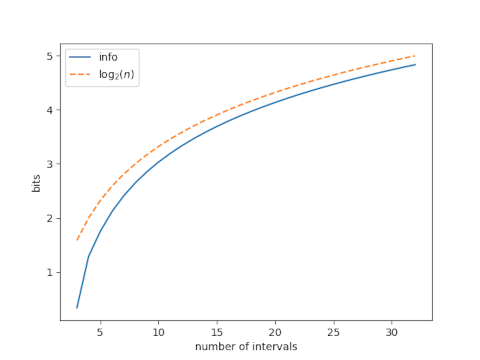The suboptimality of our bins, i.e. the difference between log2n and the information we get from n bins, drops quickly at first as n increases, then appears to plateau.

Next lets look at just the suboptimality, and increase our range of n.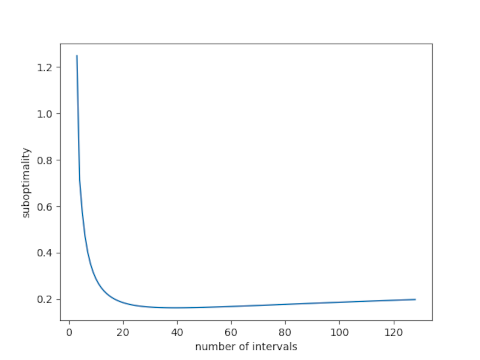This shows that the suboptimality does not approach an asymptote but instead starts to increase. The minimum at n = 36 is 0.16 bits.

# Multiserver queues with general probability distributions

Textbook presentations of queueing theory start by assuming that the time between customer arrivals and the time to serve a customer are both exponentially distributed.

Maybe these assumptions are realistic enough, but maybe not. For example, maybe there’s a cutoff on service time. If someone takes too long, you tell them there’s no more you can do and send them on their merry way. Or maybe the tails on times between arrivals are longer than those of an exponential distribution.

The default model makes other simplifying assumptions than just the arrival and service distributions. It also makes other simplifying assumptions. For example, it assumes no balking: customers are infinitely patient, willing to wait as long as necessary without giving up. Obviously this isn’t realistic in general, and yet it might be adequate when wait times are short enough, depending on the application. We will remove the exponential probability distribution assumption but keep the other assumptions.

Assuming exponential gaps between arrivals and exponential service times has a big advantage: it leads to closed-form expressions for wait times and other statistics. This model is known as a M/M/s model where the two Ms stand for “Markov” and the s stands for the number of servers. If you assume a general probability distribution on arrival gaps and service times, this is known as a G/G/s model, G for “general.”

The M/M/s model isn’t just a nice tractable model. It’s also the basis of an approximation for more general models.

Let A be a random variable modeling the time between arrivals and let S be a random variable modeling service times.

The coefficient of variation of a random variable X is its standard deviation divided by its mean. Let cA and cS be the coefficients of variation for A and S respectively. Then the expected wait time for the G/G/s model is approximately proportional to the expected wait time for the corresponding M/M/s model, and the proportionality constant is given in terms of the coefficients of variation .Note that the coefficient of variation for an exponential random variable is 1 and so the approximation is exact for exponential distributions.

How good is the approximation? You could do a simulation to check.

But if you have to do a simulation, what good is the approximation? Well, for one thing, it could help you test and debug your simulation. You might first calculate outputs for the M/M/s model, then the G/G/s approximation, and see whether your simulation results are close to these numbers. If they are, that’s a good sign.

You might use the approximation above for pencil-and-paper calculations to get a ballpark idea of what your value of s needs to be before you refine it based on simulation results. (If you refine it at all. Maybe the departure of your queueing system from M/M/s is less important than other modeling considerations.)

 See Probability, Statistics, and Queueing Theory by Arnold O. Allen.

# Queueing and Economies of Scale

If a single server is being pushed to the limit, adding a second server can drastically reduce wait times. This is true whether the server is a human serving food or a computer serving web pages. I first wrote about this here and I give more details here.

What if you add an extra server but you also add more customers? The second server still reduces the wait time, though clearly not by as much as if the work load didn’t increase.

If you increase the number of servers in proportion to the traffic, wait times will go down. In theory, wait times will approach zero as the traffic and number of servers go off to infinity together. So under ideal assumptions, you could lower wait times by scaling your number of customers and your number of servers. Alternatively, you could scale your number of servers but not as fast as your number of customers, and keep wait times constant while reducing payroll.

In theory, the ideal scale is as large as possible. Economies of scale are real, but so are dis-economies of scale. Eventually the latter overtakes the former. But that’s a matter for another post. For this post we’re only looking at an idealized model.

## Examples

As before we assume the time between customer arrivals and the time required to serve each customer is random. (Technically, a M/M/s model.)

Assume first that the ratio of the rate at which customers arrive to the rate at which they can be served is 0.8. Here’s what happens when we increase the traffic and the number of servers proportionally.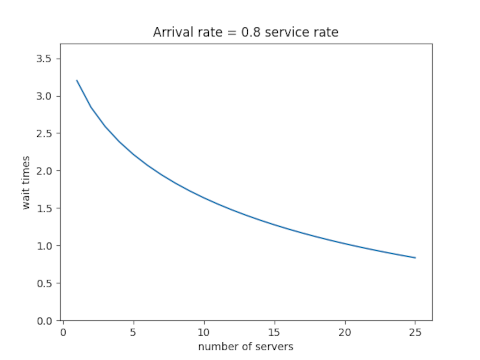Next assume that the ratio of the arrival and service rates is 0.9. Scaling the traffic and servers proportionally reduces the number of customers in line, but the wait time declines more slowly than it did when each server wasn’t so close to capacity.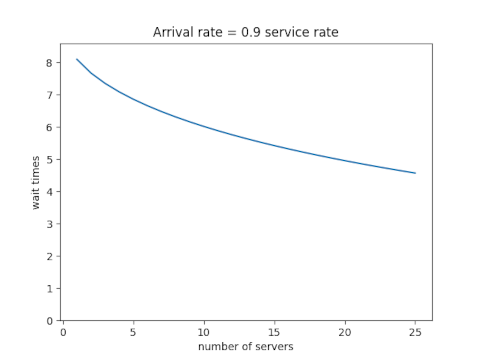Each new server helps, but each helps less than the one before. You hit diminishing return immediately.

## Equations

Let ρ be the ratio of the total arrival rate to the rate at which a single server can take care of a customer and let s be the number of servers. In the examples above, ρ was 0.8s and 0.9s. The equations below don’t assume any particular relation between ρ and s except that ρ/s must be less than 1. (If ρ/s were greater than 1, the system would not approach an equilibrium; lines would grow without bound over time.)

Define p0 as follows. (NB: that’s a Roman p, not a Greek ρ. They’re visually similar, but it’s conventional notation in queueing theory.)Then the expected wait time isBy the way, we’ve assumed the time between arrivals and the time to serve a customer are both exponentially distributed. What if they’re not? That is the subject of my next post.

# Swanson’s rule of thumb

Swanson’s rule of thumb  says that the mean of a moderately skewed probability distribution can be approximated by the weighted average of the 10th, 50th, and 90th percentile, with weights 0.3, 0.4, and 0.3 respectively. Because it is based on percentiles, the rule is robust to outliers. Swanson’s rule is used in the oil and gas industry, but I don’t believe it’s widely known outside of that sector.

This post will apply Swanson’s rule to three distributions to see how well it does. I’ll plot the bias in Swanson’s rule and skewness for three distributions families as the shape parameters vary.

First, here’s a plot for the gamma distribution with shape parameter k varying from 0.5 to 10.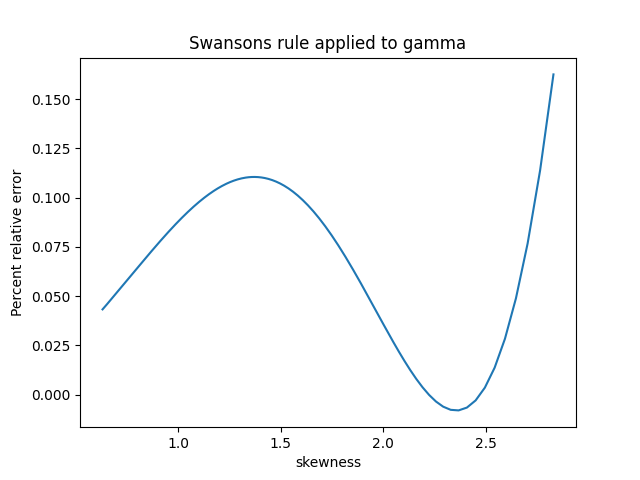The skewness of a gamma distribution is 1/√k. So unless k is close to 0, the skewness of a gamma distribution is small and the error in Swanson’s rule is very small.

For the log normal I let the shape parameter σ vary from 0.01 to 1; I didn’t use larger parameters because skewness for the log normal increases very rapidly as a function of σ. Over the range I plotted the percentage relative error in Swanson’s rule is roughly equal to the skewness.The skewness of a Pareto distribution is undefined unless the shape parameter is greater than 3. The plot below is based on shape parameters between 3.1 and 4. The skewness decreases as the shape parameter increases, asymptotically approaching 2.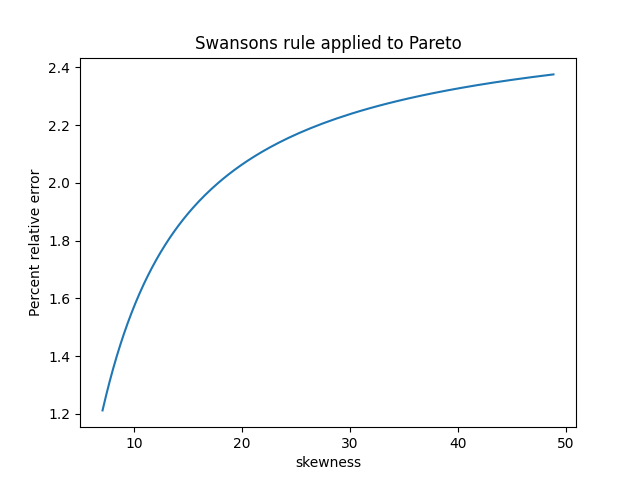Based on these examples, Swanson’s rule does indeed work pretty well when skewness is small. It works well for the gamma distribution in general because skewness is always small (unless the shape parameter k is tiny). But the log normal distribution shows that the assumption of small skewness is important.

For the Pareto distribution, Swanson’s rule works well when the shape parameter is large and the skewness is small. But this was kind of a stress test for Swanson’s rule because often the Pareto distribution is used when the skewness is large if not infinite.

 Swanson’s 30-40-30 rule. A. Hurst, G. C. Brown, R. I. Swanson. AAPG Bulletin, December 2000